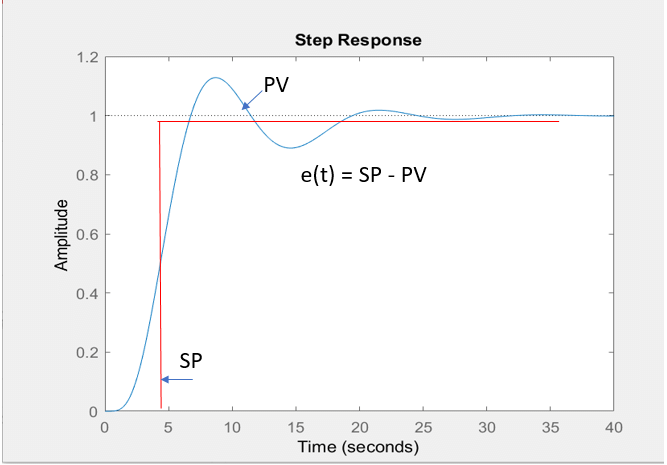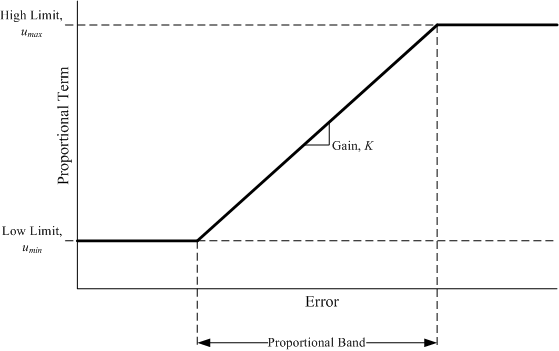Technical Article

# Proportional Gain and Proportional Band Explained

November 01, 2022 by Antonio Armenta

## Learn about proportional gain and proportional band, two key proportional control concepts, to better understand the most popular control system method in industrial automation.

Proportional control is the most popular control system method found in industrial automation. Proportional gain and proportional band are two key proportional control concepts essential to understanding this method. This article seeks to explain these concepts in simple terms.

### Proportional Control

Let us begin with the definition of proportional control (PC). A control system designed following the PC method works with a set point value and a present value. The set point is the target value for the system's output, while the present value is the latest output measurement fed back to the controller as an input.##### Figure 1. A typical response of a controlled variable in a proportional control system. Image used courtesy of H. Bryan Riley

The difference between the set point and present values is called an error. Therefore:

$$Error=Set~Point-Process~Variable$$

Or simply:

$$e=SP-PV$$

The main goal of the proportional controller is to minimize error. Ideally, the error would be zero, but that is not what happens with most real-life controlled variables (temperature, flow, pressure, torque).

Simple proportional controllers require the error to be different from zero to generate a proportionate response. This is why more advanced proportional controllers exist, incorporating additional mathematical functions into the process. However, they all rely on the proportional method to get the output as close to the set point value as possible.

#### Proportional Integral (PI) Controller

In a PI controller, mathematical integration is applied with proportional control to reduce the offset further. The integral element adjusts the controller response based on historical information about the error.

#### Proportional Derivative (PD) Controller

Mathematically, a derivative yields the slope of a curve, and that slope represents a prediction of a variable. A PD controller combines proportional control with a prediction of the error. The derivative of the error curve represents that prediction.

#### Proportional-Integral-Derivative (PID) Controller

Perhaps the most widely used form of controller in today’s applications (thanks to the benefits of incredible algorithm processing speed in even the most basic modern CPUs). Simply put, a PID controller combines all the previous functions and calibrates them with advanced algorithms.

As we have seen, all these controllers follow the PC method. Now let us take a step back and discuss gain and band.

### Proportional Gain and Proportional Band

The relationship between the response of the controller upon input of the present value of the controlled variable is expressed with the following formula:

$$P_{out}=K_p e(t)-P_0$$

• $$P_{out}$$ = proportional controller output
• $$K_p$$ = proportional gain
• $$e(t)$$ = present error = Set Point Value – Present Value
• $$P_0$$ = controller output at zero error

As you can see, this equation is the same as the straight-line equation ($$y = mx + b$$). Therefore, the controller output ($$P_{out}$$) is determined by the present error ($$e(t)$$) multiplied by the slope of the line ($$K_p$$) and a line offset ($$P_0$$).

This also explains why this method has the word “proportional” in it. It is because the controller’s output is directly proportional to the error. The gain determines that proportion or ratio.

The proportional gain is the ratio of a change in the output to a change in the input. It answers the question, how much will my output change if I change my input this much?

Let us use an example. The most popular signal transmission standard in control automation is the 4-20 mA DC range, where 4 mA represents 0%, and 20 mA is 100% scale-wise. We have a controlled system that handles inputs and outputs within this range. In this system, every time the input changes by 1 mA, the output changes by 3 mA. The gain is calculated as follows:

$$K_p=\frac{Output}{Input}$$

$$\frac{Output}{Input}=\frac{3~mA}{1~mA}$$

$$\frac{3~mA}{1~mA}=3$$

In other words, in this system, every change in output is three times as large as the change in the input.##### Figure 2. Proportional Gain and Band in the straight line equation of Proportional Control. Image used courtesy of Johnson Controls

Proportional band is another very important concept. The proportional band tells us the total range of the controlled output that can be produced within the controller’s 0% and 100% limits.

The graph above shows the same straight-line equation of proportional output or term ($$P_{out}$$) versus error. Remember that the error is simply the difference between the set point and the present values. The gain ($$K_p$$) is also shown as the slope of the straight line.

All process controllers have upper ($$u_{max}$$) and lower ($$u_{min}$$) range limits. In the transmission standard discussed before, is $$u_{min}$$ = 4 mA and $$u_{max}$$ = 20 mA. So, the proportional band tells us how much we can affect our controlled variable within the operating range of our controller. It is also known as the throttling range.

The proportional band has an inverse relation to the gain, thus, the formula to calculate it is simple:

$$PB=\frac{1}{K_p}$$

The PB is also expressed as a percentage. In our previous example, the gain of the process was 3; then, the proportional band would be 1/3 or 33.33% of the controlled output.

### PID Control Systems

PID controls are typically handled by algorithms designed by the product manufacturer. However, understanding the proper use of each proportional, integral, and derivative function can allow fine-tuning for nearly any system. The relationship between proportional gain and band is a fundamental concept of control engineering.

##### Featured image from Control Automation Online Textbook

Interested in learning more about PID controls and gain?

Articles:

Textbook: